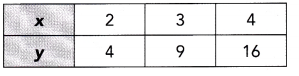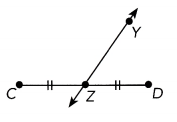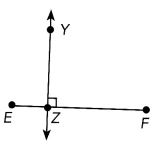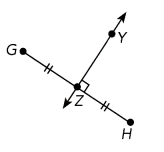# Math in Focus Grade 8 Chapter 8 Answer Key Geometric Transformations

Practice the problems of Math in Focus Grade 8 Workbook Answer Key Chapter 8 Geometric Transformations to score better marks in the exam.

## Math in Focus Grade 8 Course 3 B Chapter 8 Answer Key Geometric Transformations

### Math in Focus Grade 8 Chapter 8 Quick Check Answer Key

Question 1.
Points W and Z are reflections of each other on the x-axis. If W is the point (4, -3), what are the coordinates of Z?
Given that the points W and Z are reflections of each other on the x-axis.
The points of W are (4,-3)
The points (4,-3) are in the 4th quadrant.
The coordinates of Z are (4,3) because the reflection to the fourth quadrant in the x axis is the first quadrant. The points (4,3) lie in the first quadrant.

Question 2.
Points P (-2, 5) and Q (-2, -5) are reflections of each other in the line k. What is an equation of line k?
Given that the points are P(-2,5) and Q(-2,-5)
(x1, y1) = (-2,5)
(x2,y2) = (-2,-5)
We know that, Equation of the line formula is y2 – y1/x2 – x1
Equation of the line k = y2 – y1/x2 – x1
k = -5-(5)/-2-(-2)
k = -5-5/-2+2
k = -10/0
k = -10.

Question 3.
Points M (2, -1) and N (-2, -1) are reflections of each other in the line m. What is an equation of line m?
Given points are M(2,-1) and N(-2,-1)
Here (x1,y1) = (2,-1)
(x2,y2) = (-2,-1)
We know that, Equation of the line formula is y2 – y1/x2 – x1
equation of the line m = y2 – y1/x2 – x1
m = -1-(-1)/-2-(2)
m = -1+1/-4
m = 0/-4
Therefore m = 0

Question 4.
If a point S is the reflection of R (1,4) in the x-axis, what is the length of $$\overline{R S}$$?
Given point is R(1,4)
The point R is reflection to S(1,-4)
The distance between the RS is 8 grids.

State whether x and y are directly proportional.

Question 5.
y = 1.5x
Given equation is y = 1.5x
Suppose x,y = 2
Substitute in the above equation
2 = 1.5 × 2
2 = 3
Here x increases then y also increases so, the given equation is directly proportional.

Question 6.
y = –$$\frac{x}{2}$$
Given equation is y = -x/2
Suppose x,y = 2
Substitute in the above equation
2 = -2/2
2 = -1
Here x increases then y decreases so, it is not directly proportional.

Question 7.x = y²
We take x = 2 and y = 2
Then 2 = 2²
2 = 4
Here x increases then y decreases so, it is directly proportional.

State whether $$\overleftrightarrow{Y Z}$$ is a perpendicular bisector of the given segment. Justify your answer. If $$\overleftrightarrow{Y Z}$$ is a perpendicular bisector, name two pairs of distances that are equal.

Question 8.In the given figure CD and ZY are not perpendicular bisectors.
Perpendicular bisector means it is a line or a segment that passes through the midpoint of the segment. And any point on the perpendicular bisector is equidistant from the endpoints of the line segment.
Here the two pairs CD and ZY distances are not equal.

Question 9.